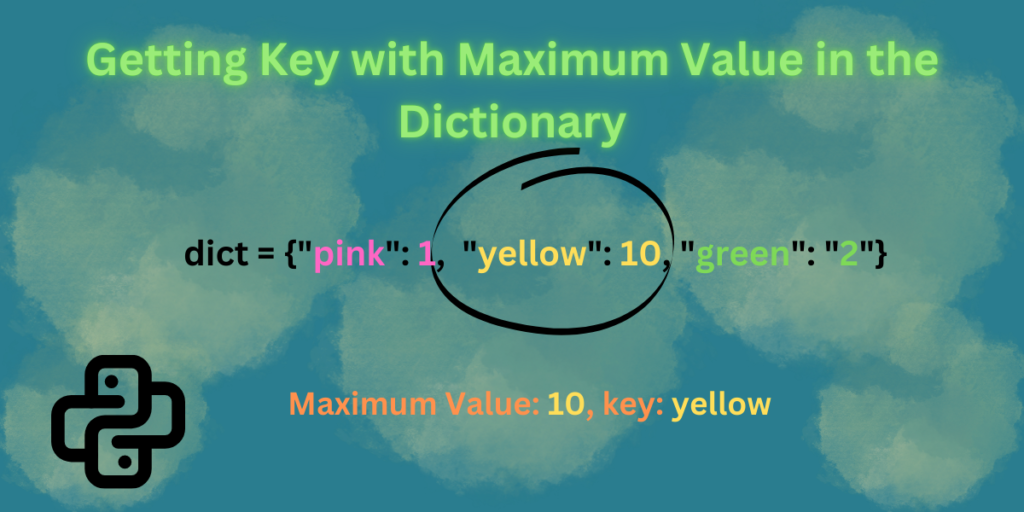# Getting Key with Maximum Value in the DictionaryA dictionary is a data structure used for storing a group of objects in python. Dictionaries have a set of keys and values, each with an associated value. In simple words, we can say the dictionary stores data in key-value pairs. A pair of curly braces `"{}"` creates a dictionary with no value.

Keys are unique within a dictionary, while values may not be. Keys are unique identifiers.

## What is a Dictionary?

The dictionary is a data structure like lists, tuples, and sets. A dictionary is used for storing data or a group of objects. Dictionary stores data in key-value pairs. In simple words, we can say the dictionary stores data in key-value pairs. A pair of curly braces `"{}"` creates a dictionary with no value. We add the key: value separated with the comma.

### Syntax:

```dict = { key: value, "key": value, "key": "value" ..... }
```

Let’s take a look at a simple example of a dictionary

```dict = {"name": "Dhushi", "age": 6, "color": "white"}

for k in dict.keys():
print(f'key: {k}')

for v in dict.values():
print(f'value: {v}')

for key, value in dict.items():
print(f'Key: {key} and value: {value}')
```
1. assigned a dictionary to variable dict with keys(name, age, color) and values(Dhushi, 6, white)
2. firstly, printing only the keys => name, age, color using the for loop and keys() method, which will return all the keys present in the dictionary
3. secondly, printing only the values=> Dhushi, 6, white using the for loop and values() method, which will return all the values present in the dictionary
4. lastly, printing keys and values using the for loop and items() method, which will return all the keys and values present in the dictionary

In the result, we see that firstly all the keys are printed using the keys() method, then all the values of the dictionary using the values() method, and lastly, we are getting both keys and values using the items() method.

You may check: For Loop with Two Variables in Python

## Using max() function

As we understand by seeing the word max, which means maximum value. So here, also max() function returns the item with the highest value. Let’s take a look at an example of how to use it.

### Example 1:

```dict = {"Dhushi": 6, "Lee": 32, "Pink": 12}

print(max(dict, key = dict.get))
```
1. assigning dictionary with key-values pair to variable dict like we normally do
2. here we are printing the key with the maximum value using `max()` function
3. use `max()` function with the key parameter set to `dict.get()` to find and return the key of the maximum value in the given dictionary

### Syntax of max() function

```max(iterable, *iterables, key, default)
```

`dict.get` is a function that returns a value for the corresponding key in dict. When `max` looking for the largest value, it uses the return value of the `key`. When we pass `dict` into `max function`, it iterates over the keys of the dictionary. `max()` returns the largest element from an iterable.

### Example 2:

In this example, we are using `zip()` function inside the max function. Python’s zip() function is defined as zip(*iterables). The zip() function is used to aggregate or combine multiple iterables.

```dict = {"Dhushi": 6, "Lee": 32, "Pink": 12}

result = max(zip(dict.values(), dict.keys()))
print(result)
```
1. assigned a dictionary with keys and values to dict variable
2. in the result variable, we are storing the maximum value key by using the `max()` function and` zip() `function
3. here, inside the zip() function, we are iterating over `dict.values()` for the values and `dict.keys()` for the keys, and from there, the max process works over both keys and values, prints the  index, which means keys here. If we put  index, then it will print the value; if we do not put anything, it will showcase both the maximum value and key.

In the result, we get the key(Lee) as it has the maximum value(32) among the other key-value pair.

## Example 3:

In this example, we will use the lambda function inside the max() function to find the key with the maximum value. Lambda is an anonymous function that can take any number of arguments.

```dict = {'Dhushi':6, 'Lee':32, 'Pink': 100}
print(max(dict, key=lambda k: dict[k]))
```
1. assigned a dictionary with keys and values to dict variable
2. here we are printing the maximum value key using the max and lambda functions inside it. The function `key` simply returns the value that should be used for ranking and `max()` returns the demanded element right away.
In this article, we learned how to create a dictionary and how we can find only keys and values using the `keys()` and `values()` method. The `items()` method is used for showing both keys-values of the dictionary. We use the `max() `function to find the maximum value in the dictionary. Inside the max function, we used the `zip` and `lambda` functions which helped us to find the keys with the maximum value.